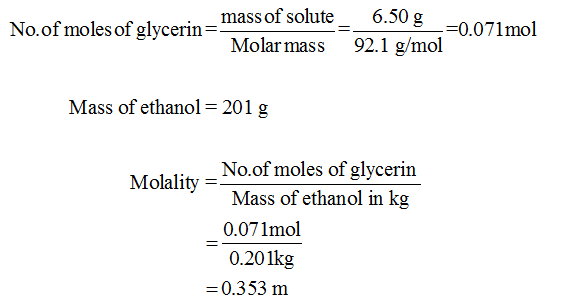# A solution is prepared by dissolving 6.50g of glycerin I'm 201g ethanol. The freezing point of the solution is? The freezing point of pure ethanol is -114.6 degrees Celsius at 1 atm. The molal- freezing point depression constant K1 for ethanol is 1.99 degrees Celsius. The molar mass of glycerin and ethanol are 92.1g/mol and 46.1 g/mol respectively

Question
2 views

A solution is prepared by dissolving 6.50g of glycerin I'm 201g ethanol. The freezing point of the solution is? The freezing point of pure ethanol is -114.6 degrees Celsius at 1 atm. The molal- freezing point depression constant K1 for ethanol is 1.99 degrees Celsius. The molar mass of glycerin and ethanol are 92.1g/mol and 46.1 g/mol respectively

check_circle

Step 1

Molality of solution can be calculated as follows:...

### Want to see the full answer?

See Solution

#### Want to see this answer and more?

Solutions are written by subject experts who are available 24/7. Questions are typically answered within 1 hour.*

See Solution
*Response times may vary by subject and question.
Tagged in